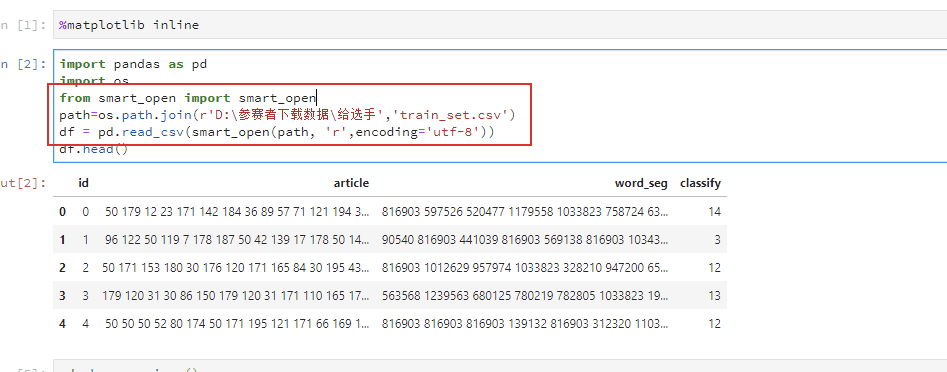2016-05-16 14:42:01 kellyseeme 阅读数 3750
• ###### Python入门视频教程

购买课程后，请扫码进入学习群，获取唐宇迪老师答疑 Python入门基础教程：python教程旨在掌握python开发的入门基础用法与其核心操作，便于大家在短时间内可以使用python进行后续的工作与学习。课程内容包括Python环境搭建（Anaconda方式）、变量、List基础结构、List索引、循环结构、判断结构、字典结构、文件处理、函数基础等。

16978 人正在学习 去看看 唐宇迪

```import os.path
import time
while os.path.getsize('messages') <1000000000:
f = open('messages','a')
f.write('this is a file/n')
f.close()

print 'file create complted'```

```#22s
start_time = time.time()
f = open('messages','r')
for i in f:
end_time = time.time()
print end_time - start_time
break
f.close()

#22s
start_time = time.time()
f = open('messages','r')
end_time = time.time()
print end_time - start_time
break
f.close()

start_time = time.time()
f = open('messages','r')
f.close()
end_time = time.time()
print end_time - start_time```

python 处理大数据文件 相关内容

2018-07-16 10:20:16 weixin_40127170 阅读数 504
• ###### Python入门视频教程

购买课程后，请扫码进入学习群，获取唐宇迪老师答疑 Python入门基础教程：python教程旨在掌握python开发的入门基础用法与其核心操作，便于大家在短时间内可以使用python进行后续的工作与学习。课程内容包括Python环境搭建（Anaconda方式）、变量、List基础结构、List索引、循环结构、判断结构、字典结构、文件处理、函数基础等。

16978 人正在学习 去看看 唐宇迪python 处理大数据文件 相关内容

2018-03-05 10:59:47 IBoyMan 阅读数 529
• ###### Python入门视频教程

购买课程后，请扫码进入学习群，获取唐宇迪老师答疑 Python入门基础教程：python教程旨在掌握python开发的入门基础用法与其核心操作，便于大家在短时间内可以使用python进行后续的工作与学习。课程内容包括Python环境搭建（Anaconda方式）、变量、List基础结构、List索引、循环结构、判断结构、字典结构、文件处理、函数基础等。

16978 人正在学习 去看看 唐宇迪
` `
``###   enumerate(ad_set,start=1)``
``###   start=1：指定索引起始值``
``````#-*- coding:utf-8 -*-
#唯一号码转家庭、非家庭
#编写者：zqm
#日期：20170810
#功能：分别找出家庭，非家庭用户，家庭的编号转jz编号，非家庭重新编号且相同编号相同
######################################################################
# 备注：
#       File_target_zhengq_temp(临时文件)是处理的文件中的政企数据
#
#
# 注意：
#######################################################################

from datetime import datetime
from sys import argv
import os

#配置文件
File_source = "/JZ_lzy.txt"

#处理文件所在的目录
dir = os.getcwd()+"/"

#要处理的文件
File_deal = argv

if File_deal.find("/") != -1:
File_deal = argv.split("/")[-1]

#临时文件即处理文件里的政企数据
File_target_zhengq_temp = dir+"政企原始数据.txt"

#政企结果文件
File_target_zhengq = dir+"zq_"+File_deal

#家庭结果文件
File_target_jiating = dir+"jt_"+File_deal

print ("程序已开始执行！！！")
print (datetime.now().strftime('%Y-%m-%d %H:%M:%S'))

dist_source = {}
list = []
dist2 = {}

with open(File_source, "r") as ff:
for line in ff:
line = line.strip().split("\t")
dist_source[line] = line

def Main_zhengqi():
with open(File_target_zhengq_temp, "w") as f_write:
with open(File_deal, "r") as ff:
for line in ff:
line = line.strip().split("\t")
if line not in dist_source:
f_write.write("\t".join(line) + "\n")

with open(File_target_zhengq_temp,"r") as ff:
for line in ff:
list.append(line)

with open(File_target_zhengq, "w") as f_write:
for line in list:
line = line.strip().split("\t")
if line in dist2:
f_write.write(str(dist2[line])+"\t"+"\t".join(line[1:])+"\n")
print("政企已完成！！！")
print(datetime.now().strftime('%Y-%m-%d %H:%M:%S'))

def Main_jiating():
with open(File_target_jiating, "w") as f_write:
with open(File_deal, "r") as ff:
for line in ff:
line = line.strip().split("\t")
if line not in dist_source:
continue
line = dist_source[line]
f_write.write("\t".join(line) + "\n")
print("家庭已完成！！！")
print(datetime.now().strftime('%Y-%m-%d %H:%M:%S'))

if __name__ == "__main__":
Main_jiating()
Main_zhengqi()
print("程序已完成！！！")
print(datetime.now().strftime('%Y-%m-%d %H:%M:%S'))``````

python 处理大数据文件 相关内容

2014-12-12 17:15:59 liqiang19910328 阅读数 470
• ###### Python入门视频教程

购买课程后，请扫码进入学习群，获取唐宇迪老师答疑 Python入门基础教程：python教程旨在掌握python开发的入门基础用法与其核心操作，便于大家在短时间内可以使用python进行后续的工作与学习。课程内容包括Python环境搭建（Anaconda方式）、变量、List基础结构、List索引、循环结构、判断结构、字典结构、文件处理、函数基础等。

16978 人正在学习 去看看 唐宇迪

XML文档的内容为

```<?xml version="1.0"?>
<data>
<country name="Liechtenstein">
<rank>1</rank>
<year>2008</year>
<gdppc>141100</gdppc>
<neighbor name="Austria" direction="E"/>
<neighbor name="Switzerland" direction="W"/>
</country>
<country name="Singapore">
<rank>4</rank>
<year>2011</year>
<gdppc>59900</gdppc>
<neighbor name="Malaysia" direction="N"/>
</country>
<country name="Panama">
<rank>68</rank>
<year>2011</year>
<gdppc>13600</gdppc>
<neighbor name="Costa Rica" direction="W"/>
<neighbor name="Colombia" direction="E"/>
</country>
</data>
```

```import xml.etree.ElementTree as ET
tree = ET.parse('country_data.xml')
root = tree.getroot()
```

```root = ET.fromstring(country_data_as_string)
```

```>>> root.tag
'data'
>>> root.attrib
{}
```

```>>> for child in root:
...   print child.tag, child.attrib
...
country {'name': 'Liechtenstein'}
country {'name': 'Singapore'}
country {'name': 'Panama'}
```

```>>> root.text
'2008'
```

python 处理大数据文件 相关内容

2019-12-04 18:02:55 zhiranyouni 阅读数 5
• ###### Python入门视频教程

购买课程后，请扫码进入学习群，获取唐宇迪老师答疑 Python入门基础教程：python教程旨在掌握python开发的入门基础用法与其核心操作，便于大家在短时间内可以使用python进行后续的工作与学习。课程内容包括Python环境搭建（Anaconda方式）、变量、List基础结构、List索引、循环结构、判断结构、字典结构、文件处理、函数基础等。

16978 人正在学习 去看看 唐宇迪

python 处理大数据文件 相关内容